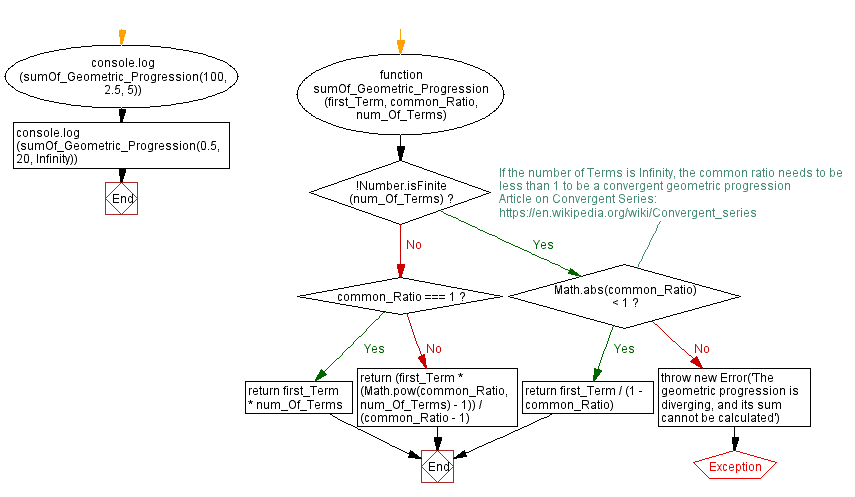# JavaScript: Sum of a geometric progression

## JavaScript Math: Exercise-67 with Solution

Write a JavaScript program to calculate the sum of a geometric progression.

From Wikipedia,
In mathematics, a geometric series is the sum of an infinite number of terms that have a constant ratio between successive terms. For example, the seriesis geometric, because each successive term can be obtained by multiplying the previous term by 1/2. In general, a geometric series is written as a + ar + ar2 + ar3 + ..., where a is the coefficient of each term and r is the common ratio between adjacent terms. The geometric series had an important role in the early development of calculus, is used throughout mathematics, and can serve as an introduction to frequently used mathematical tools such as the Taylor series, the complex Fourier series, and the matrix exponential.

Sample Data:
(100, 2.5, 5) -> 6443.75
(0.5, 20, Infinity) -> Error

Sample Solution:

HTML Code:

``````<!DOCTYPE html>
<html>
<meta charset="utf-8">
<title>JavaScript function to sum of a geometric progression</title>
<body>

</body>
</html>
```
```

JavaScript Code:

``````/**
* https://bit.ly/3gCyxWa
* first_Term: The first term of the geometric progression.
* common_Ratio: The common ratio of the geometric progression.
* num_Of_Terms: The number of terms in the progression.
*/
function sumOf_Geometric_Progression(first_Term, common_Ratio, num_Of_Terms) {
if (!Number.isFinite(num_Of_Terms)) {
/*
If the number of Terms is Infinity, the common ratio needs to be less than 1 to be a convergent geometric progression
Article on Convergent Series: https://en.wikipedia.org/wiki/Convergent_series
*/
if (Math.abs(common_Ratio) < 1) return first_Term / (1 - common_Ratio)
throw new Error('The geometric progression is diverging, and its sum cannot be calculated')
}
if (common_Ratio === 1) return first_Term * num_Of_Terms
return (first_Term * (Math.pow(common_Ratio, num_Of_Terms) - 1)) / (common_Ratio - 1)
}
console.log(sumOf_Geometric_Progression(100, 2.5, 5))
console.log(sumOf_Geometric_Progression(0.5, 20, Infinity))
```
```

Sample Output:

```6443.75
--------------------------------------------------------------------------------------
Uncaught Error: The geometric progression is diverging, and its sum cannot be calculated
at https://cdpn.io/cpe/boomboom/pen.js?key=pen.js-3a654673-3f71-908f-113a-2a3523b2059c:14
```

Flowchart:Live Demo:

See the Pen javascript-math-exercise-67 by w3resource (@w3resource) on CodePen.

Improve this sample solution and post your code through Disqus

What is the difficulty level of this exercise?

Test your Programming skills with w3resource's quiz.

﻿